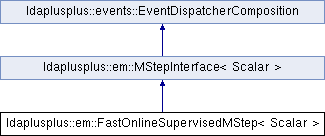LDA++
ldaplusplus::em::FastOnlineSupervisedMStep< Scalar > Class Template Reference

#include <FastOnlineSupervisedMStep.hpp>

Inheritance diagram for ldaplusplus::em::FastOnlineSupervisedMStep< Scalar >:## Public Member Functions

FastOnlineSupervisedMStep (VectorX class_weights, Scalar regularization_penalty=1e-2, size_t minibatch_size=128, Scalar eta_momentum=0.9, Scalar eta_learning_rate=0.01, Scalar beta_weight=0.9)

FastOnlineSupervisedMStep (size_t num_classes, Scalar regularization_penalty=1e-2, size_t minibatch_size=128, Scalar eta_momentum=0.9, Scalar eta_learning_rate=0.01, Scalar beta_weight=0.9)

virtual void m_step (std::shared_ptr< parameters::Parameters > parameters) override

virtual void doc_m_step (const std::shared_ptr< corpus::Document > doc, const std::shared_ptr< parameters::Parameters > v_parameters, std::shared_ptr< parameters::Parameters > m_parameters) overridePublic Member Functions inherited from ldaplusplus::events::EventDispatcherComposition
std::shared_ptr< EventDispatcherInterfaceget_event_dispatcher ()

void set_event_dispatcher (std::shared_ptr< EventDispatcherInterface > dispatcher)

## Detailed Description

### template<typename Scalar> class ldaplusplus::em::FastOnlineSupervisedMStep< Scalar >

FastOnlineSupervisedMStep is an online implementation of the fsLDA.

m_step() is called by doc_m_step() according to the minibatch_size constructor parameter thus the model parameters are updated many times in an EM step.

Each m_step() updates the $$\eta$$ parameters using an SGD with momentum update and the $$\beta$$ using the equation $$\beta_{n+1} = w_{\beta} \beta_{n} + (1-w_{\beta}) * MLE$$.

In the maximization with respect to $$\eta$$ the first order taylor approximation to the expectation of the log normalizer is used as in the FastSupervisedMStep.

## Constructor & Destructor Documentation

template<typename Scalar >
 ldaplusplus::em::FastOnlineSupervisedMStep< Scalar >::FastOnlineSupervisedMStep ( VectorX class_weights, Scalar regularization_penalty = 1e-2, size_t minibatch_size = 128, Scalar eta_momentum = 0.9, Scalar eta_learning_rate = 0.01, Scalar beta_weight = 0.9 )

Create an FastOnlineSupervisedMStep that accounts for class imbalance by weighting the classes.

Parameters
 class_weights Weights to account for class imbalance regularization_penalty The L2 penalty for the logistic regression minibatch_size After that many documents call m_step() eta_momentum The momentum for the SGD update of $$\eta$$ eta_learning_rate The learning rate for the SGD update of $$\eta$$ beta_weight The weight for the online update of $$\beta$$
template<typename Scalar >
 ldaplusplus::em::FastOnlineSupervisedMStep< Scalar >::FastOnlineSupervisedMStep ( size_t num_classes, Scalar regularization_penalty = 1e-2, size_t minibatch_size = 128, Scalar eta_momentum = 0.9, Scalar eta_learning_rate = 0.01, Scalar beta_weight = 0.9 )

Create an FastOnlineSupervisedMStep that uses uniform weights for the classes.

Parameters
 num_classes The number of classes regularization_penalty The L2 penalty for the logistic regression minibatch_size After that many documents call m_step() eta_momentum The momentum for the SGD update of $$\eta$$ eta_learning_rate The learning rate for the SGD update of $$\eta$$ beta_weight The weight for the online update of $$\beta$$

## Member Function Documentation

template<typename Scalar >
 void ldaplusplus::em::FastOnlineSupervisedMStep< Scalar >::doc_m_step ( const std::shared_ptr< corpus::Document > doc, const std::shared_ptr< parameters::Parameters > v_parameters, std::shared_ptr< parameters::Parameters > m_parameters )
overridevirtual

This function calculates all necessary parameters, that will be used for the maximazation step. And after seeing minibatch_size documents actually calls the m_step.

Parameters
 doc A single document v_parameters The variational parameters used in m-step in order to maximize model parameters m_parameters Model parameters, used as output in case of online methods

Implements ldaplusplus::em::MStepInterface< Scalar >.

template<typename Scalar >
 void ldaplusplus::em::FastOnlineSupervisedMStep< Scalar >::m_step ( std::shared_ptr< parameters::Parameters > parameters )
overridevirtual

Maximize the ELBO.This function usually changes the passed in parameters.

Parameters
 parameters Model parameters (maybe changed after call)

Implements ldaplusplus::em::MStepInterface< Scalar >.

The documentation for this class was generated from the following files: